# Oops...

This website is made for modern browsers. You are seeing this, because your browser is missing a feature or two. Please install the latest update or switch to a modern browser. See you soon.

# Divide curves

This how-to guide will focus on how to divide a curve with pattern-based rules. These rules are given by the designer within the algorithm and do not necessarily rely on any event. To see how curves can be split at intersection events, see Split curves.

The components that are introduced below output the coordinates for the division points and the curve parameters t, which can be used with Shatter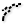Shatter (Shatter)
Curve  >  Division  >  Shatter
Shatter a curve into segments.
Inputs
Curve (C)Curve to trim
Parameters (t)Parameters to split at
Outputs
Segments (S)Shattered remains
to actually split the curve into smaller segments.

### Divide a curve in n segments (Divide Curve)

The component Divide Curve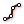Divide Curve (Divide)
Curve  >  Division  >  Divide Curve
Divide a curve into equal length segments
Inputs
Curve (C)Curve to divide
Count (N)Number of segments
Kinks (K)Split segments at kinks
Outputs
Points (P)Division points
Tangents (T)Tangent vectors at division points
Parameters (t)Parameter values at division points
will divide a curve into a number N of equal length segments. If input K is set to True, the output will also include the parameters at the kinks.
To get the parameters at the kinks only, use the component Discontinuity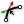Discontinuity (Disc)
Curve  >  Analysis  >  Discontinuity
Find all discontinuities along a curve.
Inputs
Curve (C)Curve to analyze
Level (L)Level of discontinuity to test for (1=C1, 2=C2, 3=Cinfinite)
Outputs
Points (P)Points at discontinuities
Parameters (t)Curve parameters at discontinuities
.
To get the parameters at the kinks only, use the component DiscontinuityDiscontinuity (Disc)
Curve  >  Analysis  >  Discontinuity
Find all discontinuities along a curve.
Inputs
Curve (C)Curve to analyze
Level (L)Level of discontinuity to test for (1=C1, 2=C2, 3=Cinfinite)
Outputs
Points (P)Points at discontinuities
Parameters (t)Curve parameters at discontinuities
.
After obtaining the curve parameters, we can use ShatterShatter (Shatter)
Curve  >  Division  >  Shatter
Shatter a curve into segments.
Inputs
Curve (C)Curve to trim
Parameters (t)Parameters to split at
Outputs
Segments (S)Shattered remains
to split the curve into several segments.

### Divide a curve by distance (Divide Distance)

The component Divide Distance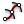Divide Distance (DivDist)
Curve  >  Division  >  Divide Distance
Divide a curve with a preset distance between points
Inputs
Curve (C)Curve to divide
Distance (D)Distance between points
Outputs
Points (P)Division points
Tangents (T)Tangent vectors at division points
Parameters (t)Parameter values at division points
will divide a curve by the distance from one division point to the next. The distance is calculated by drawing a radius around one point and setting the next point where the curve meets this radius.

### Divide a curve by length (Divide Length)

The component Divide Length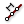Divide Length (DivLength)
Curve  >  Division  >  Divide Length
Divide a curve into segments with a preset length
Inputs
Curve (C)Curve to divide
Length (L)Length of segments
Outputs
Points (P)Division points
Tangents (T)Tangent vectors at division points
Parameters (t)Parameter values at division points
is similar to the previous one, but it uses the actual length of the curve’s segments to set the division points.

Divide Length divides the curve into n segments with the same length (except for the last one). To divide a curve into segments with varying length, we can use Evaluate Length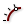Evaluate Length (Eval)
Curve  >  Analysis  >  Evaluate Length
Evaluate a curve at a certain factor along its length. Length factors can be supplied both in curve units and normalized units. Change the [N] parameter to toggle between the two modes.
Inputs
Curve (C)Curve to evaluate
Length (L)Length factor for curve evaluation
Normalized (N)If True, the Length factor is normalized (0.0 ~ 1.0)
Outputs
Point (P)Point at the specified length
Tangent (T)Tangent vector at the specified length
Parameter (t)Curve parameter at the specified length
: We set input N to False to prevent normalization and provide a list of lengths at input L. Note that the lengths that we provide to not relate to the step size (length of the segments) but to the length parameter from the start of the curve.

### Divide curve into a dash patter (Dash Pattern)

The component Dash Pattern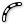Dash Pattern (Dash)
Curve  >  Division  >  Dash Pattern
Convert a curve to a dash pattern.
Inputs
Curve (C)Curve to dash
Pattern (Pt)An collection of dash and gap lengths.
Outputs
Dashes (D)Dash segments
Gaps (G)Gap segments
will divide and shatter a curve into segments given by the pattern at Pt. Output D emits the dash curves and output G the gap curves. Another way to get the dash curves would be to use Divide LengthDivide Length (DivLength)
Curve  >  Division  >  Divide Length
Divide a curve into segments with a preset length
Inputs
Curve (C)Curve to divide
Length (L)Length of segments
Outputs
Points (P)Division points
Tangents (T)Tangent vectors at division points
Parameters (t)Parameter values at division points
, ShatterShatter (Shatter)
Curve  >  Division  >  Shatter
Shatter a curve into segments.
Inputs
Curve (C)Curve to trim
Parameters (t)Parameters to split at
Outputs
Segments (S)Shattered remains
and a Cull Pattern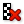Cull Pattern (Cull)
Sets  >  Sequence  >  Cull Pattern
Cull (remove) elements in a list using a repeating bit mask.
Inputs
List (L)List to cull
Cull Pattern (P)Culling pattern
Outputs
List (L)Culled list
.

The dash pattern does not have to be a single number, but can also be a list of numbers. The pattern expresses the step size and is repeated until the curve is consumed (the pattern shown below does the same splitting as the pattern inputted to Evaluate Length in the second last figure).

### Divide a curve with contour lines (Contour)

The component Contour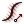Contour (Contour)
Curve  >  Division  >  Contour
Create a set of Curve contours
Inputs
Curve (C)Curve to contour
Point (P)Contour start point
Direction (N)Contour normal direction
Distance (D)Distance between contours
Outputs
Contours (C)Resulting contour points (grouped by section)
Parameters (t)Curve parameters for all contour points
is similar to Divide Distance; instead of using the distance from one point to another, the distance D (step size) is set on a guidance vector N, which starts at point P. At each step, a plane normal to the vector is generated and the division points are created where the curve meet the plane.

The component Contour (ex)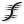Contour (ex) (Contour)
Curve  >  Division  >  Contour (ex)
Create a set of Curve contours
Inputs
Curve (C)Curve to contour
Plane (P)Base plane for contours
Offsets (O)Contour offsets from base plane (if omitted, you must specify distances instead)
Distances (D)Distances between contours (if omitted, you must specify offset instead)
Outputs
Contours (C)Resulting contour points (grouped by section)
Parameters (t)Curve parameters for all contour points
also divides a curve by contour lines, but uses a pattern instead of a fixed distance. Input P takes the base plane and then either O or D have to be provided. O defines the offsets from the base plane (so every value relates to the origin of the plane). Alternatively, we can set the Distances D between two planes, which can be a list with varying numbers. The division points are created where the curve meets the planes.

This page is open source. Edit it on GitHub or see how you can contribute.